NCERT Solutions for Class 10 Maths Chapter 12 Areas Related to Circles Ex 12.3 are part of NCERT Solutions for Class 10 Maths. Here we have given NCERT Solutions for Class 10 Maths Chapter 12 Areas Related to Circles Ex 12.3.

 Board CBSE Textbook NCERT Class Class 10 Subject Maths Chapter Chapter 12 Chapter Name Areas Related to Circles Exercise Ex 12.3 Number of Questions Solved 16 Category NCERT Solutions

## NCERT Solutions for Class 10 Maths Chapter 12 Areas Related to Circles Ex 12.3

Question 1.
Find the area of the shaded region in the given figure, if PQ = 24cm, PR = 7cm and O is the centre of the circle.
Solution: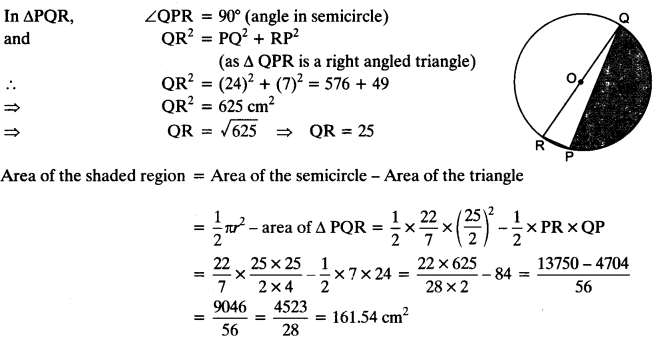Question 2.
Find the area of the shaded region in the given figure, if radii of the two concentric circles with centre O are 7 cm and 14 cm respectively and ∠AOC = 400.
Solution:
∠AOC = 40° (given)
Radius of the sector AOC = 14 cmQuestion 3.
Find the area of the shaded region in the given figure, if ABCD is a square of side 14 cm and APD and BPC are semicircles.
Solution:
ABCD is a squareGiven: side of the square = 14 cm
∴ Area of the square = (side)² = (14)² = 196 cm²
Radius of the semicircle APD = $$\frac { 1 }{ 2 }$$(side of square) = $$\frac { 1 }{ 2 }$$ x 14 = 7 cm
Area of the semicircle APD = $$\frac { 1 }{ 2 }$$ πr² = $$\frac { 1 }{ 2 }$$ × $$\frac { 22 }{ 7 }$$ × 7 × 7 = 11 × 7 = 77cm²
Similarly, area of the semicircle BPC = 77 cm²
Total area of both the semicircles = 77 + 77 = 154 cm²
Area of the shaded region = Area of square – area of both semicircles
= 196 – 154 = 42 cm²

Question 4.
Find the area of the shaded region in the figure, where a circular arc of radius 6 cm has been drawn with vertex O of an equilateral triangle OAB of side 12 cm as centre.
Solution:
Area of the equilateral triangle OABQuestion 5.
From each corner of a square of side 4 cm a quadrant of a circle of radius 1 cm is cut and also a circle of diameter 2 cm is cut as shown in the figure. Find the area of the remaining portion of the square.Solution:
Given: side of the square ABCD = 4 cm
Area of the square ABCD = 4 x 4 = 16 cm²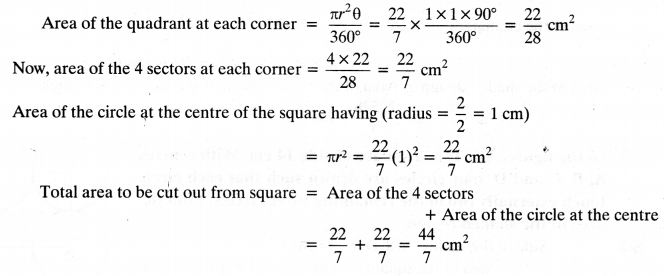∴ Area of the remaining portion = Area of the square – Area to be cut from square
= 16 – ($$\frac { 44 }{ 7 }$$) = 16 – $$\frac { 44 }{ 7 }$$
= $$\frac { 112-44 }{ 7 }$$ = $$\frac { 68 }{ 7 }$$cm²

Question 6.
In a circular table cover of the radius 32 cm, a design is formed leaving an equilateral triangle ABC in the middle as shown in the figure. Find the area of the design (shaded region).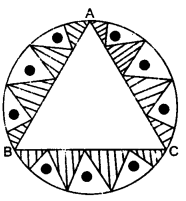Solution:
Radius of the circle(r) = 32cm
Area of the circle = πr²
= $$\frac { 22 }{ 7 }$$ × 32 × 32 = $$\frac { 22528 }{ 7 }$$cm²
∴ An equilateral triangle is formed in the circle as shown
Angle subtended by et ch side at centre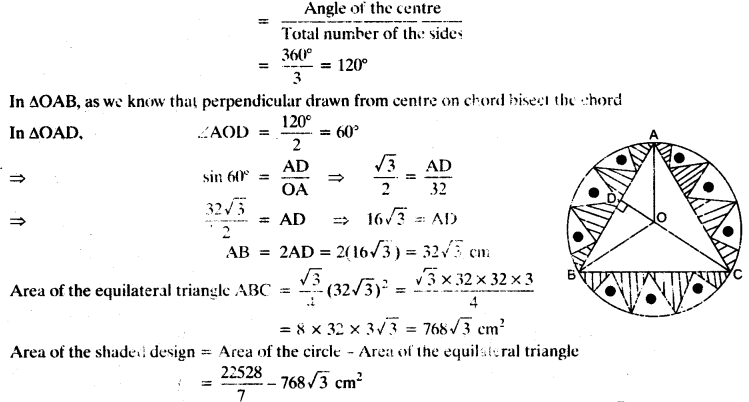Question 7.
In the figure, ABCD is a square of side 14 cm. With centres A, B, C and D, four circles are drawn such that each circle touch externally two of the remaining three circles. Find the area of the shaded region.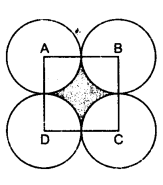Solution:
Side of the square ABCD = 14 cm
Area of the squat e = (side)² = 14 x 14 = 196²Question 8.
The given figure depicts a racing track whose left and right ends are semicircular. The distance between the two inner parallel line segments is 60 m and they are each 106 m long. If the track is 10 m wide, find: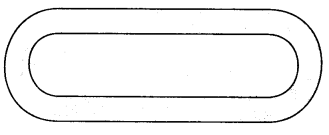(i) the distance around the track along its inner edge.
(ii) the area of the track.
Solution:Area of the tracks at both semicircular ends = 2 x 1100 = 2200 cm²
Area of the 2 rectangular portions = 2 x l x h = 2 x 106 x 10 = 2120 cm²
Total area of the track = area of the track at semicircular ends + area of the rectangular portions
= 2200 + 2120 = 4320 cm²

Question 9.
In the figure, AB and CD are two diameters of a circle (with centre O) perpendicular to each other and OD is the diameter of the smaller circle. If OA = 7 cm, find the area of the shaded region.Solution:
Given: OA = 7 cm
Radius of the semicircle ABC = OA = 7 cm
Area of the semicircle ABC = $$\frac { 1 }{ 2 }$$πr² = $$\frac { 1 }{ 2 }$$ x $$\frac { 22 }{ 7 }$$ x 7 x 7 = 11 x 7 = 77 cm²
Diameter AB = 2(OA) = 2 x 7 = 14 and OA = OC = 7 cm (radius)Question 10.
The area of an equilateral triangle ABC is 17320.5 cm². With each vertex of the triangle as centre, a circle is drawn with radius equal to half the length of the side of the triangle (see figure). Find the area of the shaded region.
(Use π = 3.14 and $$\sqrt{3}$$ = 1.73205).Solution:
Given: area of an equilateral triangle ABC = 17320.5 cm²
Let side of the triangle AB’C be ‘a’
∴ Area of the ∆ABC = $$\frac { \sqrt { 3 } }{ 4 } { a }^{ 2 }$$
$$\frac { \sqrt { 3 } }{ 4 } { a }^{ 2 }$$ = 17320.5Now,
area of the all 3 sectors = $$\frac { 3×31400 }{ 6 }$$ = 15700 cm²
∴ Area of the shaded portion = Area of the equilateral triangle
– Area of the three sectors formed at each vertex)
= 17320.5 – 15700 = 1620.5 cm²

Question 11.
On a square handkerchief, nine circular designs each of the radius 7 cm are made (see figure). Find the area of the remaining portion of the handkerchief.Solution:
Radius of the one circular design = 7 cm
Area of the one circular design = πr² = $$\frac { 22 }{ 7 }$$ x 7 x 7 = 154 cm²
Now, area of the 9 circular designs = 9 x 154 = 1386 cm²
Diameter of the circular design = 7 x 2 = 14 cm
Side of the square = 3(diameter of one circle) = 3 x 14 = 42 cm
Area of the square = 42 x 42 = 1764 cm²
Area of the remaining portion of handkerchief
= Area of the square – (Area of the 9 circular designs)
= 1764 – 1386
= 378 cm²

Question 12.
In the figure, OACB is a quadrant of a circle with centre O and radius 3.5 cm. If OD = 2 cm, find the area of the (i) quadrant OACB, (ii) shaded region.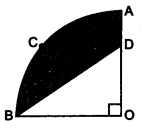Solution:(ii) OD = 2cm and OB = 3.5 cm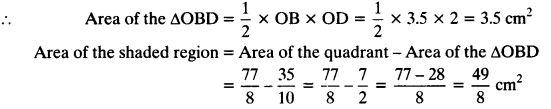Question 13.
In the figure, a square OABC is inscribed in a quadrant OPBQ. If OA = 20 cm, find the area of the shaded region. (Use re = 3.14)
Solution:
Given: side of the square OABC = OA = 20 cm
Area of the square = 20 x 20 = 400 cm²
(Diagonal of the square)² = (side of the square)² + (side of the square)² (By pythagoras theorem)
Diagonal of the square = $$\sqrt{2}$$ x (side of the square)
= $$\sqrt{2}$$ x (20) = 20$$\sqrt{2}$$cm
Radius of the quadrant of circle = Diagonal of square = 20$$\sqrt{2}$$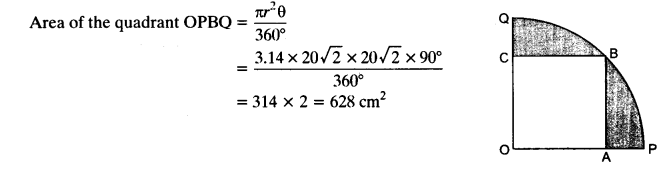Question 14.
AB and CD are respectively arcs of two concentric circles of radii 21 cm and 7 cm and centre O (see figure). If ∠AOB=30°, find the area of the shaded region.
Solution:
Given: ∠AOB = 30°
Radius of the sector AOB = 21 cmQuestion 15.
In the figure, ABC is a quadrant of a circle of radius 14 cm and a semicircle is drawn with BC as diameter. Find the area of the shaded region.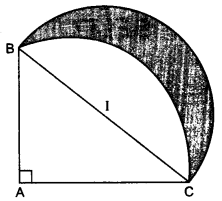Solution: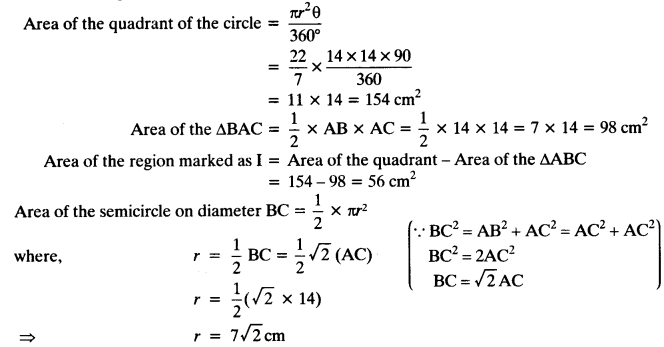Area of the shaded region = Area of the semicircular region – Area of the region I
= 154 cm² – 56 cm² = 98 cm²

Question 16.
Calculate the area of the designed region in the figure common between the two quadrants of the circles of the radius 8 cm each.
Solution:
Side of the square = 8 cm
Area of the square = 8 x 8 = 64 cm²